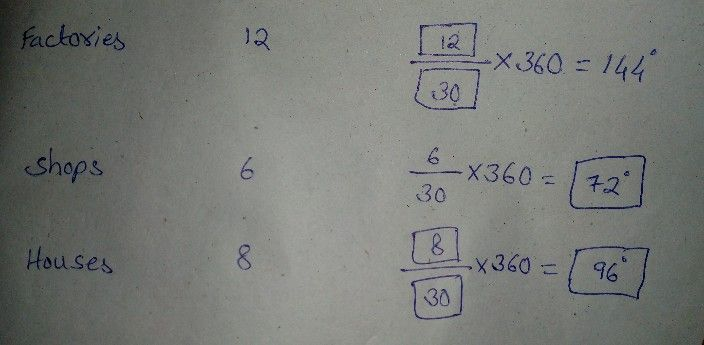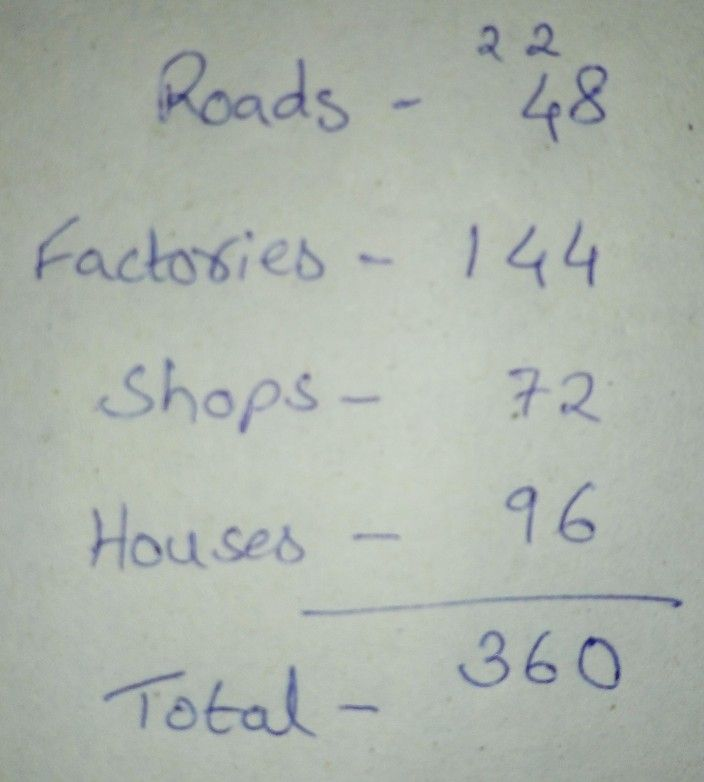Symbol
Problem$12$ $11$ The following table shows he daily supply of electricity to different places in a town. show the information by a pie diagram, measures of central angles of sectors are $l0$ decided Complete the follov ing activity to find the measures: $11$ Places Supp y of electricity Measure of central angle (Thousand units) $s\right)$ Roads $4$ $\dfrac {4} {30}\times 360=48^{°}$ Factories $12$ $-\times 360=144^{°}$ $-$ Shops $6$ $\dfrac {6} {30}\times 360=$ $\square$ Houses $8$ $-$ $-$ $\times 360=$ $→$ Total $30$
10th-13th grade
Algebra
SolutionQanda teacher - SomeoneIf you have any doubts in my answer, feel free to ask me...Student
There is no total in your answer in my question there is totalQanda teacher - Someone
Ok ok...
Wait a minute...
I will send...Student
ok
Thank youQanda teacher - SomeoneIt's ok...
We are here to help you...
Evaluate my answer and gift me some coins if you like my quick answer...Student
okQanda teacher - Someone
Thank you Rajendra...